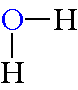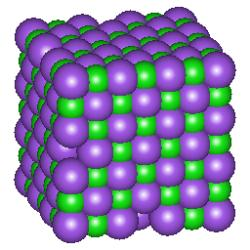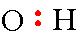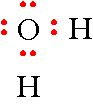# Chemical Bonding (Worksheet)

•• Richard Banks
• Boise State
$$\newcommand{\vecs}{\overset { \rightharpoonup} {\mathbf{#1}} }$$ $$\newcommand{\vecd}{\overset{-\!-\!\rightharpoonup}{\vphantom{a}\smash {#1}}}$$$$\newcommand{\id}{\mathrm{id}}$$ $$\newcommand{\Span}{\mathrm{span}}$$ $$\newcommand{\kernel}{\mathrm{null}\,}$$ $$\newcommand{\range}{\mathrm{range}\,}$$ $$\newcommand{\RealPart}{\mathrm{Re}}$$ $$\newcommand{\ImaginaryPart}{\mathrm{Im}}$$ $$\newcommand{\Argument}{\mathrm{Arg}}$$ $$\newcommand{\norm}{\| #1 \|}$$ $$\newcommand{\inner}{\langle #1, #2 \rangle}$$ $$\newcommand{\Span}{\mathrm{span}}$$ $$\newcommand{\id}{\mathrm{id}}$$ $$\newcommand{\Span}{\mathrm{span}}$$ $$\newcommand{\kernel}{\mathrm{null}\,}$$ $$\newcommand{\range}{\mathrm{range}\,}$$ $$\newcommand{\RealPart}{\mathrm{Re}}$$ $$\newcommand{\ImaginaryPart}{\mathrm{Im}}$$ $$\newcommand{\Argument}{\mathrm{Arg}}$$ $$\newcommand{\norm}{\| #1 \|}$$ $$\newcommand{\inner}{\langle #1, #2 \rangle}$$ $$\newcommand{\Span}{\mathrm{span}}$$$$\newcommand{\AA}{\unicode[.8,0]{x212B}}$$

Name: ______________________________

Section: _____________________________

Student ID#:__________________________

Work in groups on these problems. You should try to answer the questions without referring to your textbook. If you get stuck, try asking another group for help.

## Learning Objectives

1. Be able to define covalent bonds, polar covalent bonds, ionic bonds, electronegativity, dipoles, formal charge, molecular formula, structural formula and electron-dot formula.
2. Be able to recognize whether the type of bond between two atoms is covalent, polar covalent or ionic.
3. Be able to draw electron-dot formulas for simple molecules.
4. Be able to calculate the charge on an atom given the electron-dot formula.

## Bond Classifications

Chemical bonds are the attractive forces that hold atoms together in the form of compounds. A chemical bond is formed when electrons are shared between two atoms. There are three types of bonds: covalent bonds, polar covalent bonds and ionic bonds. The simplest example of bonding is demonstrated by the H2 molecule. We can see that each hydrogen atom has a single electron from the periodic table. If two hydrogen atoms come together to form a bond, then each hydrogen atom effectively has a share in both electrons and thus each resembles an inert gas structure. The two electrons that are shared are considered to be a chemical bond and can be represented with either two dots or a single dash between the two bonded atoms.

Covalent Bond: A covalent bond is formed when there is an equal sharing of electrons between two atoms. The atoms forming a covalent bond must have relatively equal attraction for the electrons. The bonds between the carbon atom and the hydrogen atoms in the compound methane CH4 are examples of covalent bonds between two different elements.

Polar covalent bond: If the atoms differ in their attraction for electrons, a polar-covalent bond will be formed. Water is an example of a molecule with this type of bond.The attraction of electrons to the nucleus of an atom is a very important property of atoms and is called electronegativity. The difference in electronegativity between bonded atoms determines the bond polarity, which is determined by the relative difference in electronegativity between two atoms that are joined together by a chemical bond. A dipole results from an unequal distribution of the electron pair in the bond between the atoms.

Ionic bonds: An ionic bond is formed when an electron is essentially transferred from one atom of a pair to the other creating ions. Sodium chloride is an example of a compound with this type of bond. These compounds exist as a crystal lattice with all the ions stacked in definite patterns. The following picture is a representation of a cubic crystal of sodium chloride. The chloride ions are represented by the purple spheres.Remember that a cation is a positively charged atom or molecule (one that has lost one or more electrons) and that an anion is a negatively charged atom or molecule (one that has gained one or more electrons). The ions are held together by electrostatic attractions. Ionic compounds have high melting points and many are soluble in water.

Most compounds that contain metals are ionic. For the purpose of this course, you may assume that all compounds that contain metals are ionic. If a compound contains the ammonium ion, it will be ionic. That is why the compound ammonium nitrate is written as NH4NO3 rather than N2H4O3. This alerts people to the fact that the compound contains the ammonium group and the nitrate group.

## Methods of Showing Formulas of Compounds

• Molecular formulas show only the type and number of atoms in a molecule.
• Structural formulas show the atoms in their correct placement in the molecule.
• Electron-dot formulas are similar to structural formulas but also include all of the non-bonding outer electrons. Knowledge of electron placement aids in understanding how molecules and elements react with one another.

## Drawing Electron-Dot Formulas

Step 1: Sum up the total number of valence electrons for the elements in the molecule. If the molecule is charged, subtract one electron for each positive charge or add one electron for each negative charge.

Step 2: Write the structure for the molecule with a pair of electrons (or a dash) between each atom. Groups of atoms will usually have the less electronegative atom surrounded by atoms having greater electronegativity. Never place a hydrogen atom in the center since it can only form one bond. Think about the valence of each atom and make sure that these valences are not exceeded except in the case of ions. Ions always have more or less bonds than the normal uncharged atom. The common valence or number of bonds formed for some common atoms are:

• H = 1
• O and S = 2
• N = 3
• C = 4
• F, Cl, Br and I = 1

Step 3: Place electrons around the outer atoms to fill their outer shells. Most atoms require eight electrons (the octet rule) so they will resemble an inert gas. Elements in Group I to Group 13 often do not follow the octet rule and have less than eight electrons in the final formula.

Step 4: Subtract the number of electrons used so far from the total calculated in Step 1 and place these remaining electrons on the central atom or atoms.

Step 5: If the central atom ends up with less than 8 electrons, then it probably forms a multiple bond with an adjacent outer atom.

Step 6: Finally you should calculate the formal charge on the atoms.

## Example 1: Methane CH4

The first electron-dot formula we shall draw will be methane, CH4.

Step 1: The first thing that you must do is to determine the number of electrons available for the formula.

• Hydrogen is a Group 1 element and thus has 1 valance electron. Since there are 4 hydrogen atoms, the total for hydrogen will be 4 electrons.
• Carbon is in Group 14 element thus has 4 outer electrons (remember that we don't use the atomic number for electron-dot formulas).

The total number of electrons for all atoms is 8.

Step 2: The next step is to determine which atom will be in the center of the molecule. Hydrogen atoms are only able to form one bond to other atoms so we need to put the carbon atom in the center.

Step 3: The next step requires drawing a "C" and "H" and place 2 electrons between these atoms.Step 4: All you have to do now is draw all of the other hydrogen atoms around the carbon atom and place 2 electrons between these hydrogen atoms and the carbon atom. This gives you a total of 8 electrons and you have drawn the electron-dot formula for methane.Step 5: A full octet. No further effort needed.

Step 6: Calculating the Formal Charges for elements in methane.

The formal charge for each hydrogen atom is calculated by the following calculation...

$f_c = 1 - \dfrac{1}{2}(2) -0 =0 \nonumber$

The first number represents the Roman Numeral over the periodic table column. The number "2" in the parentheses represents the shared electrons and there are no non-bonding electrons so the last number is zero.

The formal charge for the carbon atom is calculated by the following calculation...

$f_c = 4- \dfrac{1}{2}(8) -6 =1 \nonumber$

## Example 2: Water H2O

Then let's try a compound that has non-bonding electron pairs: water.

Step 1: The first thing that you must do is to determine the number of electrons available for the formula.

• Hydrogen is a Group 1 element and thus has 1 valance electron. Since there are 2 hydrogen atoms, the total for hydrogen will be 2 electrons.
• Oxygen is a Group 16 element thus has 6 outer electrons (remember that we don't use the atomic number for electron-dot formulas).

The total number of electrons for all atoms is 8.

Step 2: The next step is to determine which atom will be in the center of the molecule. Hydrogen atoms are only able to form one bond to other atoms so we need to put the oxygen atom in the center.

Step 3: The next thing that you must do is to draw an "O" and "H" and place 2 electrons between these atoms.Step 4: Now repeat this with the second "H". Don't worry about where these atoms are positioned around the oxygen atom, it does not matter for this representation.Step 5: Now all you have to do is place the remaining 4 electrons on the oxygen atom. The hydrogen atoms can only share in 2 electrons so don't put more electrons around them. This gives you a total of 8 electrons and you have drawn the electron-dot formula for water.Step 6: Calculating the Formal Charge for atoms in water.

The formal charge for the hydrogen atom is calculated by the following calculation...

$f_c = 1 - \dfrac{1}{2}(2) -0 =0 \nonumber$

The first number represents the Roman Numeral over the periodic table column. The number "2" in the parentheses represents the shared electrons and there are no non-bonding electrons so the last number is zero.

The formal charge for the oxygen atom is calculated by the following calculation...

$f_c = 6- \dfrac{1}{2}(2) -4 =1 \nonumber$

## Example 3: Hydroxide Ion

The next example will be an ion with a negative charge, the hydroxide ion, $$\ce{HO^{-}}$$.

Step 1: The first thing that you must do is to determine the number of electrons available for the formula?

• Hydrogen is is a Group 1 element and thus has 1 electron.
• Oxygen is a Group 16 element and thus has 6 outer electrons.
• We must add one electron for the negative charge.

The total number of electrons for both atoms including the extra electron for the negative charge is 8.

Step 2: Draw the hydrogen atom next to the oxygen atom and place a pair of electrons between the atoms.Step 3: The last thing needed to complete the formula is to place the remaining 6 electrons around the oxygen atom.Step 6: Calculating the Formal Charge for the Hydroxide Ion, $$\ce{HO^{-}}$$.

The formal charge for the hydrogen atom is calculated by the following calculation...

$f_c = 1 - \dfrac{1}{2}(2) -0 =0 \nonumber$

The first number represents the Roman Numeral over the periodic table column. The number "2" in the parentheses represents the shared electrons and there are no non-bonding electrons so the last number is zero.

The formal charge for the oxygen atom is calculated by the following calculation...

$f_c = 6- \dfrac{1}{2}(2) -6 =1 \nonumber$

## Example 4: Ammonium Ion

Now let's see what happens when we have a positive charge and draw the ammonium ion, NH4+.

Step 1: The first thing that you must do is to determine the number of electrons available for the formula?

• Hydrogen is a Group 1 element and thus has 1 electron. Since there are 4 hydrogen atoms, we have 4 electrons.
• Nitrogen is is a Group 15 element and thus has 5 outer electrons.
• We must subtract one electron for the positive charge.

The total number of electrons for all atoms including the loss of an electron for the positive charge is 8.

Steps 2-5: Draw the nitrogen atom in the center and place the 4 hydrogen atoms around it. Draw a pair of electrons between each pair. This actually finishes the formula. Notice that this structure looks exactly like the structure for methane except that the center atom is a nitrogen atom. When two structures have identical electron-dot formulas, they are considered to be isoelectronic.Step 5:

The formal charge for each of the atoms is calculated by the following calculation:

$f_c = 1 - \dfrac{1}{2}(2) -0 =0 \nonumber$

The first number represents the Roman Numeral over the periodic table column. The number "2" in the parentheses represents the shared electrons and there are no non-bonding electrons so the last number is zero.

The formal charge for the nitrogen atom is calculated by the following calculation:

$f_c = 5- \dfrac{1}{2}(8) -6 = +1 \nonumber$

## Example 5: Molecular Oxygen

Finally, let's draw a compound that requires multiple bonds and draw the formula for oxygen, O2.

Step 1: The first thing that you must do is to determine the number of electrons available for the formula?

• Oxygen is a Group 16 element and thus has 6 outer electrons...since there are 2 oxygen atoms, there will be a total of 12 electrons.

Step 2: Draw the 2 oxygen atoms with a pair of electrons between them.Step 3: The next thing that you must do is to draw the remaining electrons in pairs around each of the oxygen atoms giving neither atom more than 8 total electrons.Step 4: The oxygen atom on the right has 8 electrons but the oxygen atom on the left has only 6 electrons. The only way to correct this problem is to create a multiple bond between the oxygen atoms. Take a pair of electrons from the oxygen atom on the right and place this pair between the 2 atoms.Step 6: Calculating the Formal Charges for elements in methane.

The formal charge for each oxygen atom is calculated by the following calculation...

$f_c = 6 - \dfrac{1}{2}(2) - 4 = 0 \nonumber$

The first number represents the Roman Numeral over the periodic table column. The number "2" in the parentheses represents the shared electrons and there are no non-bonding electrons so the last number is zero.

## Q1

1. Define a covalent bond.
2. Define a polar-covalent bond.
3. Define an ionic bond.

## Q2

1. Define electronegativity. Why does electronegativity increase going from left to right in a row in the periodic table?
2. Why does electronegativity increase going from bottom to top in a column in the periodic table?

## Q3

Describe the type of bond represented by the dash for each of the following compounds using the definitions in Q1. Consider only the atoms to which the dash is directly connected.

• H–H
• H–CH3
• H–NH2
• Na–ONa
• Na–Br
• K–OH
• H–OH
• H–Br
• F–CH3

## Q4

Draw the electron-dot formulas for the following compounds or ions and calculate the formal charges for every atom.

• HF
• H2S
• F-
• Br+
• N2
• HCN
• NC-

This page titled Chemical Bonding (Worksheet) is shared under a CC BY-NC-SA 4.0 license and was authored, remixed, and/or curated by Richard Banks.